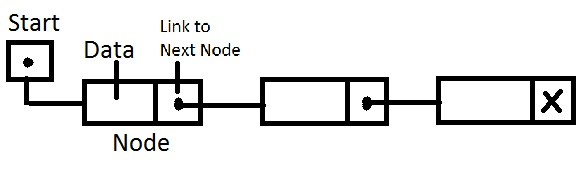Linked List in C- Insert Node at Start of the Linked List

## Linked List in C- Insert Node at Start of the Linked List :

Linked List is a data structure which is used to store and retrieve data. Consider the following image.A linked list consist of a head(start in the image) and a series of nodes. The head is the linked list points to the first node of the linked list. A node consist of data and a pointer variable which can point to next node. Usually the pointer variable is named next or link for better understanding.i.e next points to next node of the linked list. The pointer variable next of last node stores NULL which means that it is the end of linked list.

Source Code :

```#include<stdio.h>
//Declared as global variable since it is accessed by multiple functions

struct node
{
//Structure named node to store integer in variable data and a pointer variable which can store address location of next node
int data;
struct node *next;
//Pointer variable next is a self referential pointer which stores the address of structure node
};

void insert(int data);
//Function prototype for funtion insert()

void insert(int data)
{
//This function will insert a new node to the start of the linked list

struct node *temp = (struct node*)malloc(sizeof(struct node));
//malloc is used to allocate the memory dynamically
//Pointer variable temp is stored in heap
//A memory block(size of structre node) is created and a pointer variable temp is made to point to that memory location

temp->data=data;
//Store the integer data obtained from the function insert to the member variable data of newly created node

//Make newly created node point to address location where head is pointing to

//Make head pointer point to newly created node
}

void print()
{
//This funtion is used to print the data in each of the node in the linked list
//Process of moving from one node to another node is called as traversing

//Use a new pointer variable temp
//Do not use the pointer variable head directly. If we use it, the address location    of the first node in the linked list will be lost

printf("\n\nList:");
while(temp!=NULL)
{
//Linked list is traversed from one node to another and the data in each node is printed
printf("\n%d ",temp->data);

temp=temp->next;
//Pointer variable temp will initially point to first node in the linked list
//temp=temp->next will make the pointer variable temp to point to next node in the linked list
}
}

int main()
{
insert(1);
print();
//Insert the data 1 to the linked list and print the linked list

insert(2);
print();
//Insert the data 2 to the linked list and print the linked list

insert(3);
print();
//Insert the data 3 to the linked list and print the linked list

return 0;
}```

Output :

```List:
1